# Arithmetic progression sum of arithmetic progression

Definition: an Arithmetic progression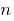is a numerical sequence, each member of which, starting from the second, equal to the previous member, to which is added the same number.

Definition: the Difference of the arithmetic progressionis constant for the sequence number, which is added to each member.— increasing arithmetic progression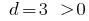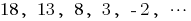— decreasing arithmetic progression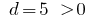arithmetic progressionthe difference of the arithmetic progression

## Characteristic properties arithmetico progression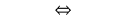Property: Any member of an arithmetic progression, starting with the second lorny average arithmetic poperechnogo nastepnego member and Vice versa, if you specified velstelt, then the sequence is an arithmetic progression.

## Formula of nth member of arithmetic progression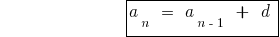## Formula sum of first n members of an arithmetic progression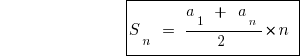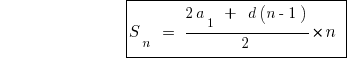## Plan the tasks for progression

1. Everything that is said in the speech tasks (members progress their amount, etc.) expressed through the first term and the difference of progression.
2. Make the equation (or system of equations) according to the problem. In the case when the task of the transition from a geometric progression to an arithmetic progression and Vice versa, to form equations of the normally used characteristic properties of progressions.
Tags:
Chapter:
Versions in other languages:
Share with friends: